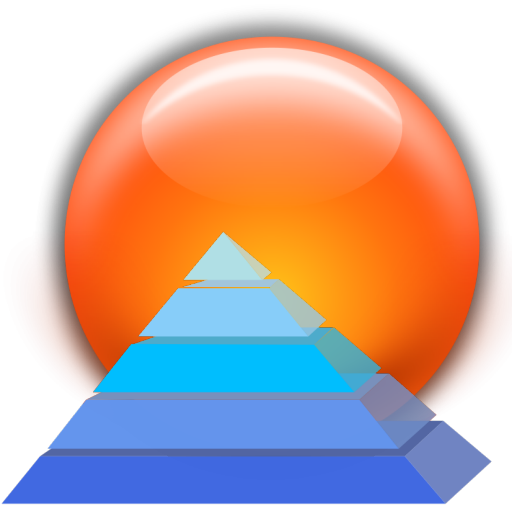# Area And Volume

Version 3.0
1000 Tomans
5
K
Active installs
Tools
Category
474
KB
Size

This is an Area and Volume Calculator.
Area Calculator: You are able to calculate the area for the most important geometric figures. You can calculate the area of circle, ellipse, rectangle, square, trapezoid, triangle and etc.
Volume Calculator: You are able to calculate the volume for the most important geometric objects. You can calculate the volume of cone, cube, cylinder, sphere and etc.